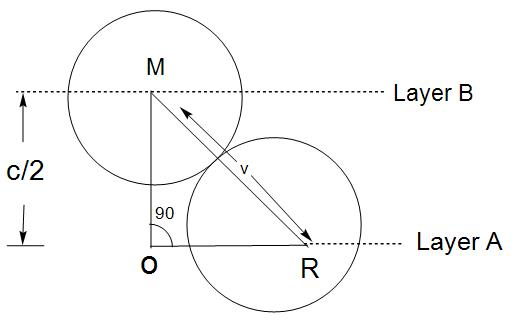QuestionAnswers

# What is the maximum number of layers of atoms in close-packed planes that will lie within two imaginary parallel planes having a distance between them as $13\sqrt{\frac{2}{3}}r$ (r is the radius of an atom) in the copper crystal (fcc)?[ Consider the atoms to be within the parallel planes if their centres are on or within the two parallel planes.]

Hint: Unit cells are basically the smallest repeating atoms in a crystal. There are different types of unit cell like body centred unit cell represented as BCC, face centred unit cell (FCC). FCC is the face centred cubic unit cell, which is having coordination number 12 and also contains 4 atoms per unit cell.

- We will first draw the diagram:- Here, we know that the distance given in between parallel planes is $\frac{C}{2}$$\frac{C}{2}$\begin{align} & \left( OM \right)+\left( RO \right)={{\left( MR \right)}^{2}} \\ & \left( \frac{C}{2} \right)+\left( \frac{2r}{\sqrt{3}} \right)={{\left( 2r \right)}^{2}} \\ \end{align}
- Now, by solving this equation we get :
\begin{align} & {{\left( \frac{C}{2} \right)}^{2}}=\frac{8{{r}^{2}}}{3} \\ & C=4r\sqrt{\frac{2}{3}} \\ \end{align}
Now, we have to find the height C,
$H(C)=4r\sqrt{\frac{2}{3}}$
- We can also write this equation as:
$or,\text{ }H(C)=2a\sqrt{\frac{2}{3}}$
- Now, the distance between two layers that is A and B= $\frac{C}{2}=\sqrt{\frac{8}{3}}(r)=\sqrt{\frac{2}{3}}(2r)$
- The given distance between two imaginary plane=$13\sqrt{\frac{2}{3}}(r)$
Now, let K number of imaginary planes=
\begin{align} & K\times \frac{\sqrt{2}}{\sqrt{3}}\left( r \right) \\ & =13\sqrt{\frac{2}{3}}\left( r \right) \\ & K\approx 6.5 \\ & K=7 \\ \end{align}

Hence, we can conclude that the maximum number of layers of atoms in close-packed planes will be 7.

Note: We must not get confused in terms of FCC and BCC. FCC is face centred cubic unit cell, it contains four atoms per unit cell. Whereas, BCC is a body centred unit cell, it contains 2 atoms per unit cell.